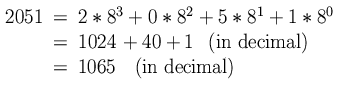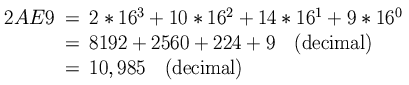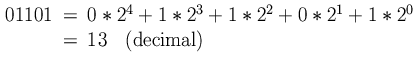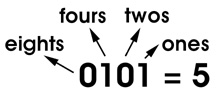<-- Back to Previous Page TOC Next Section --># Section 2.4: Binary Numbers

Now we know more than we ever wanted to know about how often to store numbers in order to digitally represent a signal. But surely speed isn’t the only thing that matters. What about size? In this section, we’ll tell you something about how big those numbers are and what they "look" like.

All digital information is stored as binary numbers. A binary number is simply a way of representing our regular old numbers as a list, or sequence, of zeros and ones. This is also called a base-2 representation.

In order to explain things in base 2, let’s think for a minute about those ordinary numbers that we use. You know that we use a decimal representation of numbers. This means that we write numbers as finite sequences whose symbols are taken from a collection of ten symbols: our digits 0, 1, 2, 3, 4, 5, 6, 7, 8, and 9.

A number is really a shorthand for an arithmetic expression. For example:Now we bet you can see a pattern here. For every place in the number, we just multiply by a higher power of ten. Representing a number in base-8, say (which is called an octal representation), would mean that we would only use eight distinct symbols, and multiply those by powers of 8 (instead of powers of 10). For example (in base 8), the number 2,051 means:There is even hexadecimal notation in which we work in base 16, so we need 16 symbols. These are our usual 0 through 9, augmented by A, B, C, D, E, and F (counting for 10 through 15). So, in base 16 we might write:But back to binary: this is what we use for digital (that is, computer) representations. With only two symbols, 0 and 1, numbers look like this:Each of the places is called a bit for (Binary digIT). The leftmost bit is called the most significant bit (MSB), and the rightmost is the least significant bit (because the digit in the leftmost position, the highest power of 2, makes the most significant contribution to the total value represented by the number, while the rightmost makes the least significant contribution). If the digit at a given bit is equal to 1, we say it is set. We also label the bits by what power of 2 they represent.

How many different numbers can we represent with 4 bits? There are sixteen such combinations, and here they are, along with their octal, decimal, and hexadecimal counterparts:

 Binary Octal Decimal Hexadecimal 0000 00 00 00 0001 01 01 01 0010 02 02 02 0011 03 03 03 0100 04 04 04 0101 05 05 05 0110 06 06 06 0111 07 07 07 1000 10 08 08 1001 11 09 09 1010 12 10 0A 1011 13 11 0B 1100 14 12 0C 1101 15 13 0D 1110 16 14 0E 1111 17 15 0F

Table 2.1  Number base chart.

Some mathematicians and philosophers argue that the reason we use base 10 is that we have ten fingers (digits)—those extraterrestrials we met in Chapter 1 who hear light might also have an extra finger or toe (or sqlurmphragk or something), and to them the millennial year might be the year 1559 (in base 11)! Boy, that will just shock them right out of their fxrmmp!qts!

What’s important to keep in mind is that it doesn’t much matter which system we use; they’re all pretty much equivalent (1010 base 2 = 10 base 10 = 12 base 8 = A base 16, and so on). We pick numeric bases for convenience: binary systems are useful for switches and logical systems, and computers are essentially composed of lots of switches.Figure 2.12  A four-bit binary number (called a nibble). Sixteen is the biggest decimal number we can represent in 4 bits.

## Numbering Those Bits

Consider the binary number 0101 from Figure 2.12. It has 4 bits, numbered 0 to 3 (computers generally count from 0 instead of 1). The rightmost bit (bit zero, the LSB) is the "ones" bit. If it is set (equal to 1), we add 1 to our number. The next bit is the "twos" bit, and if set adds 2 to our number. Next comes the "fours" bit, and finally the "eights" bit, which, when set, add 4 and 8 to our number, respectively. So another way of thinking of the binary number 0101 would be to say that we have zero eights, one four, zero twos, and one 1.

 Bit number 2Bit number Bit values 0 20 1 1 21 2 2 22 4 3 23 8

Table 2.2  Bits and their values. Note that every bit in base 2 is the next power of 2. More generally, every place in a base-n system is n raised to that place number.

Don’t worry about remembering the value of each bit. There’s a simple trick: to find the value of a bit, just raise it to its bit number (and remember to start counting at 0!). What would be the value of bit 4 in a five-bit number? If you get confused about this concept, just remember that this is simply what you learned in grade school with base 10 (the 1s column, the 10s column, the 100s column, and so on).Applet 2.4
Binary counter

This applet sonifies, or lets you hear a binary counter. Each of the 8 bits is assigned to a different note in the harmonic series (pitches that are integer multiples of the fundamental pitch, corresponding to the spectral components of a periodic waveform). As the binary counter counts, it turns the notes on and off depending on whether or not the bit is set.

The crucial question is, though, how many different numbers can we represent with four bits? Or more important, how many different numbers can we represent with n bits? That will determine the resolution of our data (and for sound, how accurately we can record minute amplitude changes). Look back at Table 2.1: using all possible combinations of the 4 bits, we were able to represent sixteen numbers (but notice that, starting at 0, they only go to 15). What if we only used 2 bits? How about 16?

Again, thinking of the analogy with base 10, how many numbers can we represent in three "places" (0 through 999 is the answer, or 1,000 different numbers). A more general question is this: for a given base, how many values can be represented with n places (can’t call them bits unless it’s binary)?

The answer is the base to the nth power.

The largest number we can represent (since we need 0) is the base to the nth power minus 1. For example, the largest number we can represent in binary with n bits is 2n – 1.

 Number of bits 2Number of bits Number of numbers 8 28 256 16 216 65,536 24 224 16,777,216 32 232 4,294,967,296

Table 2.3 How many numbers can an n-bit number represent? They get big pretty fast, don’t they? In fact, by definition, these numbers get big exponentially as the number of bits increases linearly (like we discussed when we talked about pitch and loudness perception in Section 1.2).

Modern computers use 64-bit numbers! If 232 is over 4 billion, try to imagine what 264 is (232 x 232). It’s a number almost unimaginably large. As an example, if you counted very fast, say five times a second, from 0 to this number, it would take you over 18 quintillion years to get there (you’d need the lifetimes of several solar systems to accomplish this).

OK, we’ve got that figured out, right? Now back to sound. Remember that a sample is essentially a "snapshot" of the instantaneous amplitude of a sound, and that snapshot is stored as a number. What sort of number are we talking about? 3? 0.00000000017? 16,000,000,126? The answer depends on how accurately we capture the sound and how much space we have to store our data.

 <-- Back to Previous Page Next Section -->